Maths-
General
Easy

Question

# 𝑉𝑒𝑟𝑖𝑓𝑦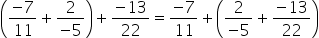Hint:

## The correct answer is: verified.

### To verifyExpress the rational numbers with positive denominator by multiplying with -1.Here,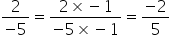Consider the LHS part, we have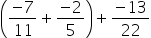…(i)Determine the LCM of the denominators of rational numbers inside the brackets.LCM of 11 and 5 = 55On multiplying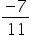with 5, we get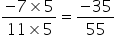On multiplying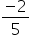with 11, we get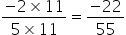On adding, we get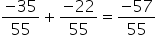On substituting this in (i), we get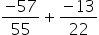Determine the LCM of the denominators of rational numbers.LCM of 55 and 22 = 110On multiplying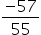with 2, we get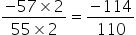On multiplying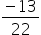with 5, we get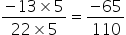On adding, we get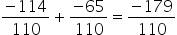Thus we have LHS to be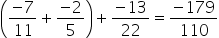Consider the RHS part, we have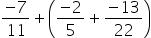…(ii)Determine the LCM of the denominators of rational numbers inside the brackets.LCM of 5 and 22 = 110On multiplyingwith 22, we get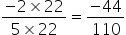On multiplyingwith 5, we getOn adding, we get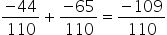On substituting this in (ii), we get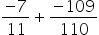Determine the LCM of the denominators of rational numbers.LCM of 11 and 110 = 110On multiplyingwith 10, we get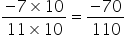On multiplying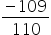with 1, we get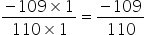On adding, we get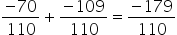Thus we have RHS to be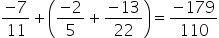So we get LHS = RHS =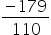Hence verified.#### With Turito Foundation.#### Get an Expert Advice From Turito.